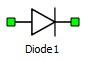# Diode

This reference section describes the properties and features of the Diode converter component of Typhoon HIL's software.

Important: It is recommended to consider using existing components before incorporating a Diode component if possible. Please consult our Knowledgebase article on the recommended uses of the Diode component and how to gain access to it.

A block diagram and input parameters for a diode are given in Table 1.

Table 1. Diode component in Schematic Editor
component component dialog window component parametersDiode• Use non-ideal switches (if disabled, ideal switch model is used)
• Snubber type (available if ideal switch is used)

Maximal weight = 1

There are two implementations available for the diode: modeled as an ideal switch or as a non-ideal switch (Figure 1 and Figure 2).

If an ideal switch model is used, diode is modeled as a short circuit when conducting and an open circuit when not conducting. In this case, a parallel snubber circuit can be defined. It can be a resistance or a series connection of a resistor and capacitor. These options are shown in Figure 1. Weight of diode modeled as an ideal switch is 1.Figure 1. R-C snubber option in case of ideal switch model of a diode.

When a Use non-ideal switch option is chosen a diode is modeled as a small inductance while conducting and a small capacitances while not-conducting. Weight of diode modeled as an ideal switch is 0. Non-ideal switch models are addressing a different resource called Non-ideal switches.The parameter Resistance (Figure 2) and the simulation step are defining the actual inductance and capacitance values according to the following equations:

$L=Ts\bullet R$

Where L and C are the calculated inductance and capacitance, Ts is the simulation step of electrical part of the model, R is the set resistance value.Figure 2. Property window of a diode when modeled as a non-ideal switch
Note: Diode component is available through special toolbox, which can be provided on request.